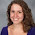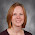Thursday, August 11, 2016

Geometry Scope and Sequence

I recently posted my algebra 2 scope and sequence.  I am also teaching geometry this year, so I figure I might as well post that too.  I am using standards based grading.  I number my objects as #.#  The first number is the unit number and the second number is the objective number within that unit.  The first year I did this, I just numbered from about 1 to 120.  It made making changes to the list more difficult.  Now when I need to add or subtract a topic, I don't need to renumber all of the objectives, just the ones in the same unit.

I would love feedback on this list.  Our students take geometry after algebra 1 and 2.  The course seems very easy and I feel like I need more rigour in the class.  Please share your list on your blog and leave a comment here so I can check it out.

Geometry Part 1
Chapter 1: Tools of Geometry
1.1 Use basic geometry terms and notation including points, lines and planes
1.2 Use basic postulates of geometry
1.3 Name segments and rays
1.4 Recognize parallel and skew lines and planes
1.5 Find the lengths of segments using the segment addition postulate and the midpoint on a number line
1.6 Name, measure, and classify angles
1.7 Find measures of angles using the angle addition postulate
1.8 Identify angle pairs
1.9 Use perpendicular bisectors and angle bisectors
1.10 Find distance on the coordinate plane
1.11 Find the midpoint or endpoint of a segment on the coordinate plane
1.12 Find perimeter or circumference
1.13 Find area of basic and composite shapes

Chapter 2: Reasoning and Proof
2.1 Use conditional statements including the hypothesis, conclusion, and counterexample
2.2 Use the converse of a conditional statement
2.3 Write the negation, inverse, and contrapositive of a conditional statement
2.4 Use indirect reasoning (proof by contradiction)
2.5 Write a biconditional or identify the parts of a biconditional
2.6 Use the law of detachment
2.7 Use the law of syllogism
2.8 Use properties of congruence
2.9 Use theorems about angles to prove congruence including vertical angle theorem, right angle theorem, linear pair theorem, congruent supplements theorem, and congruent complements theorem

Chapter 3: Parallel and Perpendicular Lines
3.1 Identify angles formed by two lines and transversal
3.2 Use properties of parallel lines including proofs and finding angle measures
3.3 Use theorems relating parallel and perpendicular lines
3.4 Use the triangle angle-sum theorem and classify triangles
3.5 Use the triangle exterior angle theorem
3.6 Classifying and naming polygons
3.7 Use the polygon angle-sum theorem
3.8 Use the polygon exterior angle angle-sum theorem
3.9 Graph lines
3.10 Write equations of lines
3.11 Determine if lines are parallel and write equations of parallel lines
3.12 Determine if lines are perpendicular and write equations of perpendicular lines

Chapter 4:  Congruent Triangles
4.1 Use congruent figures and their corresponding parts
4.2 Prove two triangles are congruent by using the SSS and SAS postulates
4.3 Prove two triangles are congruent by using the ASA and AAS postulates
4.4 Prove triangles are congruent using the HL theorem
4.5 Use triangle congruence and CPCTC to prove that parts of two triangles are congruent
4.6 Use and apply properties of isosceles and equilateral triangles
4.7 Using overlapping triangles in proofs
4.8 Using two pairs of congruent triangles

Chapter 5: Relationships within Triangles
5.1 Use the perpendicular bisector theorem
5.2 Use the angle bisector theorem
5.3 Identify properties of medians and altitudes of triangles
5.4 Use inequalities involving angles of triangles
5.5 Use inequalities involving sides of triangles

6.1 Define and classify special types of quadrilaterals
6.2 Use relationships among sides and angles of parallelograms
6.3 Use relationships involving diagonals of parallelograms or transversals
6.4 Determine whether a quadrilateral is a parallelogram
6.5 Use properties of diagonals of rhombuses and rectangles
6.6 Determine whether a parallelogram is a rhombus or a rectangle
6.7 Use properties of trapezoids
6.8 Use properties of kites
6.9 Classify quadrilaterals given coordinates or find missing coordinates of a quadrilateral

Geometry Part 2
Chapter 7:  Similarity
7.1 Write ratios and solve proportions
7.2 Identify and use similar polygons
7.3 Use properties of triangle midsegments
7.4 Use and apply AA, SAS, and SSS similarity
7.5 Find and use relationships in similar right triangles
7.6 Use the side-splitter theorem
7.7 Use the triangle-angle-bisector theorem

Chapter 8:  Right Triangles and Trigonometry
8.1 Use the Pythagorean theorem
8.2 Use the converse of the Pythagorean theorem
8.3 Use properties of 45-45-90 triangles
8.4 Use properties of 30-60-90 triangles
8.5 Use sine, cosine, and tangent ratios to determine side lengths in triangles
8.6 Use inverse trigonometry functions to determine angle measures in triangle
8.7 Use angles or elevation and depression to solve problems

Chapter 12:  Circles
12.1 Use the radius-tangent relationship
12.2 Use the relationship between two tangents from one point
12.3 Find the measures of central angles and arcs
12.4 Find the circumference and arc length
12.5 Use congruent chords, arcs, and central angles
12.6 Use properties of lines through the center of a circle
12.7 Find the measure of an inscribed angle
12.8 Find the measure of an angle formed by a tangent and a chord
12.9 Find the measures of angles formed by 2 chords
12.10 Find the measures of angles formed by a tangent and a secant, 2 secants, or 2 tangents
12.11 Find the lengths of segments associated with 2 chords
12.12 Find the lengths of segments associated with a tangent and a secant or 2 secants
12.13 Write the equation of a circle
12.14 Find the center and the radius of a circle and graph a circle

Chapter 10:  Area
10.1 Find the area of a parallelogram
10.2 Find the area of a triangle
10.3 Find the area of a trapezoid
10.4 Find the area of a rhombus or a kite
10.5 Find the area of regular polygons - trig not required
10.6 Find the perimeters and areas of similar figures
10.7 Find the area of regular polygons using trigonometry
10.8 Find the areas of circles, sectors, and segments of circles
10.9 Find probability using area

Chapter 11:  Surface Area and Volume
11.1 Identify parts of a polyhedron
11.2 Find the lateral and surface area of a prism
11.3 Find the lateral and surface area of a cylinder
11.4 Find the lateral and surface area of a pyramid
11.5 Find the lateral and surface area of a cone
11.6 Find the volume of a prism
11.7 Find the volume of a cylinder
11.8 Find the volume of a pyramid
11.9 Find the volume of a cone
11.10 Find the surface area of a sphere
11.11 Find the volume of a sphere
11.12 Find relationships between the ratios of the areas and volumes of similar solids

Chapter 9:  Transformations
9.1 Identify isometries
9.2 Find translation images of figures
9.3 Find reflection images of figures
9.4 Identify rotation images
9.5 Identify types (reflectional or rotational) symmetry
9.6 Find scale factors and graph dilation images
9.7 Use a composition of reflections
9.8 Use glide reflections
9.9 Identify transformations and symmetries in tessellations

Chapter 13:  Constructions
13.1:  Given a segment, construct a segment congruent to the given segment
13.2:  Given an angle, construct an angle congruent to the given angle
13.3:  Given an angle, construct the bisector of the angle
13.4:  Given a segment, construct the perpendicular bisector of the segment
13.5: Given a point on a line, construct the perpendicular to the line at the given point
13.6: Given a point outside a line, construct the perpendicular to the line from the given point
13.7: Given a point outside a line, construct the parallel to the line through the given point

1.1.